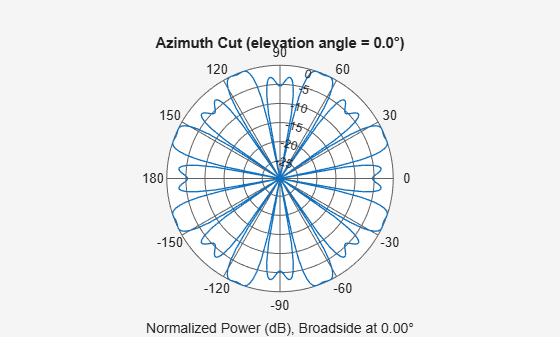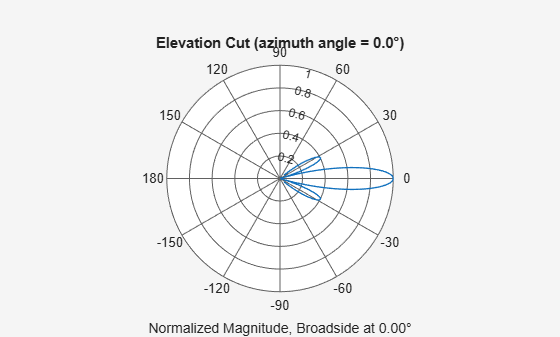Documentation

# phased.ConformalArray

Conformal array

## Description

The `ConformalArray` object constructs a conformal array. A conformal array can have elements in any position pointing in any direction.

To compute the response for each element in the array for specified directions:

1. Define and set up your conformal array. See Construction.

2. Call `step` to compute the response according to the properties of `phased.ConformalArray`. The behavior of `step` is specific to each object in the toolbox.

### Note

Starting in R2016b, instead of using the `step` method to perform the operation defined by the System object™, you can call the object with arguments, as if it were a function. For example, ```y = step(obj,x)``` and `y = obj(x)` perform equivalent operations.

## Construction

`H = phased.ConformalArray` creates a conformal array System object, `H`. The object models a conformal array formed with identical sensor elements.

`H = phased.ConformalArray(Name,Value)` creates object, `H`, with each specified property Name set to the specified Value. You can specify additional name-value pair arguments in any order as (`Name1`,`Value1`,...,`NameN`,`ValueN`).

`H = phased.ConformalArray(POS,NV,Name,Value)` creates a conformal array object, `H`, with the `ElementPosition` property set to `POS`, the `ElementNormal` property set to `NV`, and other specified property Names set to the specified Values. `POS` and `NV` are value-only arguments. When specifying a value-only argument, specify all preceding value-only arguments. You can specify name-value arguments in any order.

## Properties

 `Element` Element of array Specify the element of the sensor array as a handle. The element must be an element object in the `phased` package. Default: Isotropic antenna element with default properties `ElementPosition` Element positions `ElementPosition` specifies the positions of the elements in the conformal array. `ElementPosition` must be a 3-by-N matrix, where N indicates the number of elements in the conformal array. Each column of `ElementPosition` represents the position, in the form `[x; y; z]` (in meters), of a single element in the local coordinate system of the array. The local coordinate system has its origin at an arbitrary point. The default value of this property represents a single element at the origin of the local coordinate system. Default: `[0; 0; 0]` `ElementNormal` Element normal directions `ElementNormal` specifies the normal directions of the elements in the conformal array. Angle units are degrees. The value assigned to `ElementNormal` must be either a 2-by-N matrix or a 2-by-1 column vector. The variable N indicates the number of elements in the array. If the value of `ElementNormal` is a matrix, each column specifies the normal direction of the corresponding element in the form `[azimuth;elevation]` with respect to the local coordinate system. The local coordinate system aligns the positive x-axis with the direction normal to the conformal array. If the value of `ElementNormal` is a 2-by-1 column vector, it specifies the same pointing direction for all elements in the array. You can use the `ElementPosition` and `ElementNormal` properties to represent any arrangement in which pairs of elements differ by certain transformations. The transformations can combine translation, azimuth rotation, and elevation rotation. However, you cannot use transformations that require rotation about the normal. Default: `[0; 0]` `Taper` Element taper or weighting Element tapering or weighting, specified as a complex-valued scalar, 1-by-N row vector, or N-by-1 column vector. Weights are applied to each element in the sensor array. N is the number of elements along in the array as determined by the size of the `ElementPosition` property. If the `Taper` parameter is a scalar, the same taper value is applied to all elements. If the value of `Taper` is a vector, each taper values is applied to the corresponding element. Default: 1

## Methods

 collectPlaneWave Simulate received plane waves directivity Directivity of conformal array getElementNormal Normal vector to array elements getElementPosition Positions of array elements getNumElements Number of elements in array getTaper Array element tapers isPolarizationCapable Polarization capability pattern Plot conformal array pattern patternAzimuth Plot conformal array directivity or pattern versus azimuth patternElevation Plot conformal array array directivity or pattern versus elevation plotResponse Plot response pattern of array step Output responses of array elements viewArray View array geometry
Common to All System Objects
`release`

Allow System object property value changes

## Examples

expand all

Using the ConformalArray System object, construct an 8-element uniform circular array (UCA) of isotropic antenna elements. Plot a normalized azimuth power pattern at 0 degrees elevation. Assume the operating frequency is 1 GHz and the wave propagation speed is the speed of light.

```N = 8; azang = (0:N-1)*360/N-180; sCA = phased.ConformalArray(... 'ElementPosition',[cosd(azang);sind(azang);zeros(1,N)],... 'ElementNormal',[azang;zeros(1,N)]); fc = 1e9; c = physconst('LightSpeed'); pattern(sCA,fc,[-180:180],0,... 'PropagationSpeed',c,'Type','powerdb',... 'CoordinateSystem','polar')```Construct a 31-element acoustic uniform circular sonar array (UCA) using the ConformalArray System object. Assume the array is one meter in diameter. Using the `ElevationAngles` parameter, restrict the display to +/-40 degrees in 0.1 degree increments. Assume the operating frequency is 4 kHz. A typical value for the speed of sound in seawater is 1500.0 m/s.

Construct the array

```N = 31; theta = (0:N-1)*360/N-180; Radius = 0.5; sMic = phased.OmnidirectionalMicrophoneElement(... 'FrequencyRange',[0,10000],'BackBaffled',true); sArray = phased.ConformalArray('Element',sMic,... 'ElementPosition',Radius*[zeros(1,N);cosd(theta);sind(theta)],... 'ElementNormal',[ones(1,N);zeros(1,N)]);```

Plot the magnitude pattern

```fc = 4000; c = 1500.0; pattern(sArray,fc,0,[-40:0.1:40],... 'PropagationSpeed',c,... 'CoordinateSystem','polar',... 'Type','efield')```Plot the directivity pattern

```pattern(sArray,fc,0,[-40:0.1:40],... 'PropagationSpeed',c,... 'CoordinateSystem','polar',... 'Type','directivity')```## References

 Josefsson, L. and P. Persson. Conformal Array Antenna Theory and Design. Piscataway, NJ: IEEE Press, 2006.

 Van Trees, H. Optimum Array Processing. New York: Wiley-Interscience, 2002.